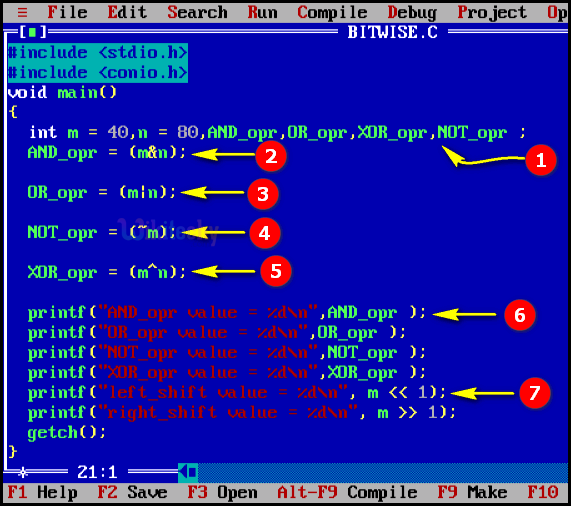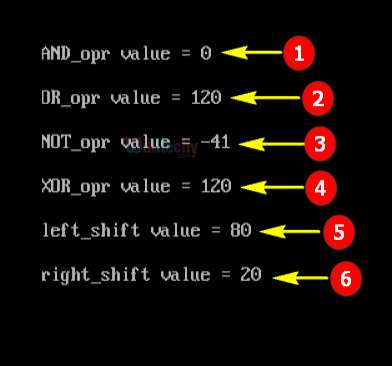# Bitwise Operation in CLearn C - C tutorial - Bitwise operation in c - C examples - C programs

## Bitwise Operation in C - Definition and Usage

• In C-programming the bitwise operators are used to perform bit operations.
• That is the decimal values are converted into binary values which are the sequence of bits and bit wise operators work on these bits.
• C provides six operators for bit manipulation. They are
• & (bitwise AND),
• | (bitwise OR),
• ~ (bitwise NOT),
• ^ (bitwise XOR),
• << (bitwise left shift) and
• >> (bitwise right shift).## Bitwise AND operator

• The Bitwise And operator denoted by &
• Take two operends and the result of bitwise AND is 1 if the corresponding bits of two operands is 1.
• If either bit of an operand is 0, the result of corresponding bit is evaluated to 0.

## Bitwise OR operator

• The Bitwise OR operator denoted by |
• Take two operends and the result of bitwise OR is 1 if atleast one of the corresponding bit of two operands is 1.

## Bitwise NOT operator

• The Bitwise NOT operator denoted by ~
• It is an unary operator
• Take only one operand and the result is complement of the operand which means changes bit 1 to 0 and 0 to 1

## Bitwise XOR operator

• The Bitwise XOR operator denoted by ^
• Take two operands and the result of bitwise XOR operator is 1 if the corresponding bits of two operands are opposite to each other

## Bitwise Left Shift operator.

• It denoted by <<
• It shifts all the bits towards left by certain number of bits which is specified by user.

## Bitwise Right Shift operator.

• It denoted by >>
• It shifts all the bits towards right by certain number of bits which is specified by user.

Operators in C

## C Code - ExplanationC Program to Reverse Bits of a Number
1. Here in this statement we initialize the value of the variable “m=40 and n=80” and defined the variables AND_opr, OR_opr , XOR_opr & NOT_opr .
2. In this statement we are using the bitwise logical operator AND (&) it works in such a way as multiplication operation, here the first value will be converted into binary format. For example, here we have now on checking each digit with their binary value the common values are set as”1” remaining are set as “0” , so you get the output as “00000000” which is the decimal value of “0” that value will be store in the variable AND_opr .
3. In this statement we are using the bitwise logical operator OR(|) and it works such a way as addition operation, here the first value will be converted into binary format .We have m=40 binary value as “00101000” and n=”80” binary value as “01010000” , now on checking each digits with their value if any digits is “1” it sets as ”1” ,if both the digits are common means it sets as “0” ,so you get the output as “01111000” which is the decimal value of “120” that value will be store in the variable OR_opr.
4. In this statement we are using the bitwise logical operator NOT(~) it works in such a way like the first value will be converted into binary format and it takes the 2’s complement for the value that will be store in this variable NOT_opr .
5. In this statement we are using the bitwise logical operator XOR(^) it works in such a way like ,the first value will be converted into binary format .Here we have m=40 binary value as “00101000” and n=”80” binary value as “01010000” now on checking each digits with their value if any digits is “1” means it sets as”1” ,if both the digits are “0” (or) “1” means it sets as “0” .so you get the output as “01111000” and the decimal value “68” that value will be store in the variable OR_opr..
6. Here we are using the printf statement for the output value to display your console window.
7. This printf statement we print the output of the bitwise left shift value of “m” at one position. And the bitwise left shift operator works like in this format for example the binary value of the “m” will be left shifted “1” times and the output decimal value as “20”.

## Sample Output - Programming Examples1. Here in this output the AND operation variables “m” (value=40;Binary value = ) and “n” (value=80) has been shown with its output result as “0” .
2. Here in this output the OR operation for the variable “m” (value=40) and “n” (value=80) has been shown with its output result as “120” .
3. Here in this output the NOT operation for the variable “m” (value=40) and “n” (value=80) has been shown with its output result as “-41” .
4. Here in this output the XOR operation for the variable “m” (value=40) and “n” (value=80) has been shown with its output result as “120” .
5. Here in this output the Left shift operation for the variable “m” (value=40) has been shown with its output result as “80” .
6. Here in this output the Right shift operation for the variable “m” (value=40) has been shown with its output result as “20” .